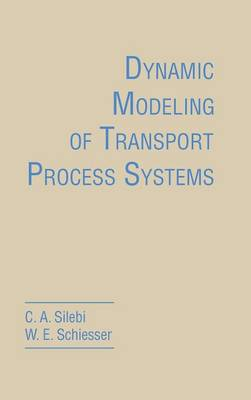•# Dynamic Modeling of Transport Process Systems (Hardback)

(author), (author)
£56.99
Hardback 518 Pages / Published: 22/05/1992
• We can order this

Usually dispatched within 3 weeks

This book presents a methodology for the development and computer implementation of dynamic models for transport process systems. Rather than developing the general equations of transport phenomena, it develops the equations required specifically for each new example application. These equations are generally of two types: ordinary differential equations (ODEs) and partial differential equations (PDEs) for which time is an independent variable. The computer-based methodology presented is general purpose and can be applied to most applications requiring the numerical integration of initial-value ODEs/PDEs. A set of approximately two hundred applications of ODEs and PDEs developed by the authors are listed in Appendix 8.

Publisher: Elsevier Science Publishing Co Inc
ISBN: 9780126434200
Number of pages: 518
Weight: 910 g
Dimensions: 229 x 152 x 33 mm